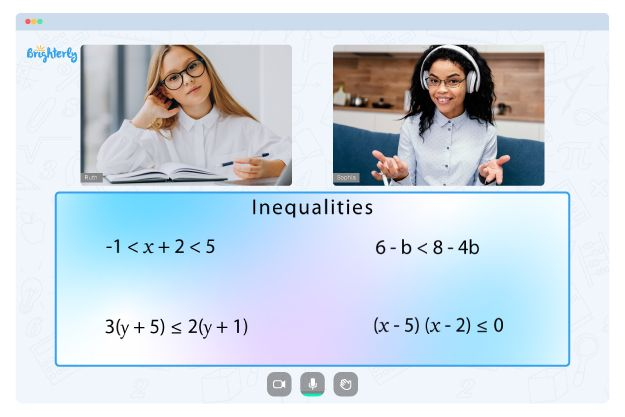# One Step Inequalities Worksheet

Students learn how to plot inequality graphs on an XY plane. You can represent inequalities graphically in 2-dimensional space (a two-variable chart), just like representing inequalities on the number line. In both cases, you can solve the inequality as an equation and test their points after solving them.

For the two variables, the answer is a line, not a single point. The line separates the inequality-satisfying area from the other region in the XY-graph.

Though the concept of inequality may be complex, solving one step inequalities worksheet resources is vital in making students confident in their abilities. One-step inequalities are similar to one-step equations. However, in contrast to the equations in which an answer is a single number, the answers are several numbers in inequalities.

## Free One-step Inequalities Worksheet

Free, downloadable, and printable one variable inequality worksheet resources are critical to helping your students learn and quickly solve one-step graph inequalities. You can solve these inequalities in one step involving one variable. Solving the one-step inequalities worksheet makes learning seamless since the resources are easy to understand.

Math for Kids

Is Your Child Struggling With Math?
1:1 Online Math TutoringThe relatable design of the solving one-step inequalities worksheet is unsurprising since top professionals design them. The design of the sheets comprises colorful and real-life objects.

You can download the printable version of the one step inequalities worksheet answers from the Brighterly website. With these materials, your kids can learn independently and continuously.### One Step Inequalities Worksheet PDF

Solving One-Step Inequalities Worksheets### One Step Inequalities Worksheet PDF

One Step Inequalities Worksheet### One Step Inequalities Worksheet PDF

Solving One Step Inequalities Worksheet### One Step Inequalities Worksheet PDF

Troubles with Inequalities?• Does your child struggle to grasp the basics of inequalities?
• Try lessons with an online tutor.

Is your child having difficulties with understanding inequalities? An online tutor could provide the necessary support.